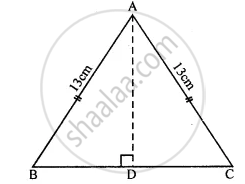# One of the Equal Sides of an Isosceles Triangle is 13 Cm and Its Perimeter is 50 Cm. Find the Area of the Triangle. - Mathematics

Sum

One of the equal sides of an isosceles triangle is 13 cm and its perimeter is 50 cm. Find the area of the triangle.

#### Solution

In isosceles ∆ABC
AB = AC = 13 cm But perimeter = 50 cm∴ BC = 50 - (13 + 13) cm

= 50 - 26 = 24 cm

∴ AD = DC = 24/2 = 12 cm.

In right ΔABD,

AB2 = AD2 + BD2  (Pythagoras Therorem)

(13)^2 = "AD"^2 + (12)^2

⇒ 169 = "AD"^2 + 144

⇒ "AD"^2 = 169 - 144

= 25 = (5)2

Now area of ΔABC = 1/2  "Base" xx "Altitude"

= 1/2 xx "BC" xx "AD"

= 1/2 xx 24 xx 5 = 60 cm2

Is there an error in this question or solution?
Chapter 20: Area of a Trapezium and a Polygon - Exercise 20 (A) [Page 224]

#### APPEARS IN

Selina Concise Mathematics Class 8 ICSE
Chapter 20 Area of a Trapezium and a Polygon
Exercise 20 (A) | Q 11 | Page 224
Share